$\newcommand{\xv}{\mathbf{x}} \newcommand{\Xv}{\mathbf{X}} \newcommand{\yv}{\mathbf{y}} \newcommand{\Yv}{\mathbf{Y}} \newcommand{\zv}{\mathbf{z}} \newcommand{\av}{\mathbf{a}} \newcommand{\Wv}{\mathbf{W}} \newcommand{\wv}{\mathbf{w}} \newcommand{\gv}{\mathbf{g}} \newcommand{\Hv}{\mathbf{H}} \newcommand{\dv}{\mathbf{d}} \newcommand{\Vv}{\mathbf{V}} \newcommand{\vv}{\mathbf{v}} \newcommand{\tv}{\mathbf{t}} \newcommand{\Tv}{\mathbf{T}} \newcommand{\zv}{\mathbf{z}} \newcommand{\Zv}{\mathbf{Z}} \newcommand{\muv}{\boldsymbol{\mu}} \newcommand{\sigmav}{\boldsymbol{\sigma}} \newcommand{\phiv}{\boldsymbol{\phi}} \newcommand{\Phiv}{\boldsymbol{\Phi}} \newcommand{\Sigmav}{\boldsymbol{\Sigma}} \newcommand{\Lambdav}{\boldsymbol{\Lambda}} \newcommand{\half}{\frac{1}{2}} \newcommand{\argmax}{\underset{#1}{\operatorname{argmax}}} \newcommand{\argmin}{\underset{#1}{\operatorname{argmin}}} \newcommand{\dimensionbar}{\underset{#1}{\operatorname{|}}}$

### The Scaled Part¶

The first derivative of an error function with respect to the parameters of your model tells you which direction in the parameter space to proceed to reduce the error function. But how far do you go? So far we have just taken a small step by subtracting a small constant times the derivative from our current parameter values.

If we are in the vicinity of a minimum of the error function, we could do what Newton did...approximate the function at the current parameter value with a parabola and solve for the minimum of the parabola. Use this as the next guess at a good parameter value. If the error function is quadratic in the parameter, then we jump to the true minimum immediately.

How would you fit a parabola to a function at a particular value of $x$? We can derive a way to do this using a truncated Taylor series (google that) to approximate the function about a value of $x$. See Taylor Series approximation, newton's method and optimization by Suzanna Sia and Taylor Series at Wolfram Mathworld starting at equation 29.

$$f(x+\Delta x) \approx \hat{f}(x+\Delta x) = f(x) + f'(x) \Delta x + \frac{1}{2} f''(x) \Delta x^2 +$$

Now we want to know what value of $\Delta x$ minimizes $\hat{f}(x+\Delta x)$. So take its derivative and set equal to zero.

\begin{align*} \frac{d \hat{f}(x+\Delta x)}{d\Delta x} &= f'(x) + \frac{1}{2} 2 f''(x) \Delta x\\ & = f'(x) + f''(x) \Delta x \end{align*}

Setting equal to zero we get

\begin{align*} 0 &= f'(x) + f''(x) \Delta x\\ \Delta x &= -\frac{f'(x)}{f''(x)} \end{align*}

Now we can update our guess for $x$ by adding $\Delta x$ to it. Then, fit a new parabola at the new value of $x$, calculate $\Delta x$, and update $x$ again. Actually, the last equation above does the parabola approximation and calculation of $\Delta x$.

Here is a simple example. Say we want to find the minimum of

$$f(x) = 2 x^4 + 3 x^3 + 3$$

To calculate

\begin{align*} \Delta x &= -\frac{f'(x)}{f''(x)} \end{align*}

we need the function's first and second derivatives. The are

\begin{align*} f'(x) &= 8 x^3 + 9 x^2\\ f''(x) &= 24 x^2 + 18 x \end{align*}

All together now, in python!

In :
import numpy as np
import matplotlib.pyplot as plt

import IPython.display as ipd  # for display and clear_output
import time  # for sleep

In :
def f(x):
return 2 * x**4 + 3 * x**3 + 3

def df(x):
return 8 * x**3 + 9 * x**2

def ddf(x):
return 24 * x**2 + 18*x

def taylorf(x, dx):
return f(x) + df(x) * dx + 0.5 * ddf(x) * dx**2

In :
# negated

def f(x):
return - (2 * x**4 + 3 * x**3 + 3)

def df(x):
return -(8 * x**3 + 9 * x**2)

def ddf(x):
return -(24 * x**2 + 18*x)

def taylorf(x, dx):
return f(x) + df(x) * dx + 0.5 * ddf(x) * dx**2

In :
x = np.random.uniform(-2, 1)  # first guess at minimum

xs = np.linspace(-2, 1, num=100)

fig = plt.figure()

dxs = np.linspace(-0.5, 0.5, num=100)

for rep in range(10):

time.sleep(1)

plt.clf()
plt.plot(xs, f(xs))
plt.grid('on')
plt.plot(x + dxs, taylorf(x, dxs), 'g-', linewidth=5, alpha=0.4)
plt.plot(x, f(x), 'ro')

# x = x - df(x) / float(ddf(x))

y0, y1 = plt.ylim()
plt.plot([x, x], [y0, y1], 'r--')

x = x - df(x) / ddf(x)

plt.plot(x, f(x), 'go')
plt.text(x, (y0 + y1) * 0.5, f'{x:.4f}', color='r')
plt.legend(('$f(x)$','$\hat{f}(x)$'))

ipd.clear_output(wait=True)
ipd.display(fig)

ipd.clear_output(wait=True)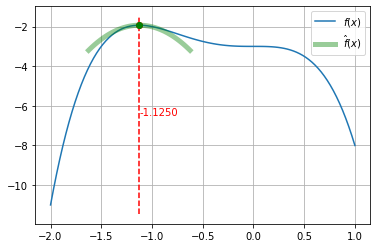This has all been for a function $f(x)$ of a single, scalar variable $x$. To minimize a squared error function for a neural network, $x$ will consist of all the weights of the neural network. If all of the weights are collected into the vector $\wv$, then the first derivative of the squared error function, $f$, with respect to the weight vector, $\wv$, is a vector of derivatives like $\frac{\partial f}{\partial w_{i}}$. This is usually written as the gradient

$$\nabla_{\wv} f = \left (\frac{\partial f}{\partial w_{1}}, \frac{\partial f}{\partial w_{2}}, \ldots, \frac{\partial f}{\partial w_{n}} \right ).$$

The second derivative will be $n\times n$ matrix of values like $\frac{\partial^2 f}{\partial w_i \partial w_j}$, usually written as the Hessian

$$\nabla^2_{\wv} f = \begin{pmatrix} \frac{\partial^2 f}{\partial w_1 \partial w_1} & \frac{\partial^2 f}{\partial w_1 \partial w_2} & \cdots \frac{\partial^2 f}{\partial w_1 \partial w_n}\\ \frac{\partial^2 f}{\partial w_2 \partial w_1} & \frac{\partial^2 f}{\partial w_2 \partial w_2} & \cdots \frac{\partial^2 f}{\partial w_2 \partial w_n}\\ \vdots \\ \frac{\partial^2 f}{\partial w_n \partial w_1} & \frac{\partial^2 f}{\partial w_n \partial w_2} & \cdots \frac{\partial^2 f}{\partial w_n \partial w_n} \end{pmatrix}$$

It is often impractical to construct and use the Hessian. We will consider ways to approximate the product of the Hessian and a matrix as part of the Scaled Conjugate Gradient algorithm.

### The Conjugate Part¶

Let $E(\wv)$ be the error function (mean square error over training samples) we wish to minimize by finding the best $\wv$. Steepest descent will find new $\wv$ by minimizing $E(\wv)$ in successive directions $\dv_0, \dv_1, \ldots$ for which $\dv_i^T \dv_j = 0$ for $i \neq j$. In other words, the search directions are orthogonal to each other, resulting in a zig-zag pattern of steps, some of which are in the same directions.

Another problem with orthogonal directions is that forcing the second direction, for example, to be orthogonal to the first will not be in the direction of the minimum unless the error function is quadratic and its contours are circles.

We would rather choose a new direction based on the previous ones and on the curvature, or second derivative, of the error function at the current $\wv$. This is the idea behind conjugate gradient methods.

The Scaled Conjugate Gradient (SCG) algorithm, Efficient Training of Feed-Forward Neural Networks, by Moller, combines conjugate gradient directions with a local, quadratic approximation to the error function and solving for the new value of $\wv$ that would minimize the quadratic function. A number of additional steps are taken to improve the quadratic approximation.

## optimizers.py¶

The three optimization algorithms discussed so far,

are implemented for you in optimizers.py which you can extract from optimizers.tar after downloading it.

Each is illustrated here as they search for the minimum of the following 3-dimensional bowl.

In :
import numpy as np
import matplotlib.pyplot as plt

import IPython.display as ipd  # for display and clear_output
import time  # for sleep

In :
from mpl_toolkits.mplot3d import Axes3D
from matplotlib.colors import LightSource

# x will be set at top level, so a global variable
# x will be row vector

def parabola(xmin, s):
d = x.reshape(-1, 1) - xmin
return d.T @ s @ d

d = x.reshape(-1, 1) - xmin
return 2 * (s @ d).reshape(-1)  # must be row vector

In :
center = np.array([5, 5]).reshape(2, 1)
S = np.array([[5, 3], [3, 5]])

n = 20
xs = np.linspace(0, 10, n)
ys = np.linspace(0, 10, n)
X,Y = np.meshgrid(xs, ys)
both = np.vstack((X.flat, Y.flat)).T
nall = n * n

Z = np.zeros(nall)
for i in range(nall):
x = both[i:i + 1, :]
Z[i] = parabola(center, S)
Z = Z.reshape(n, n)

# see https://matplotlib.org/3.1.0/gallery/mplot3d/surface3d.html
fig = plt.figure(figsize=(8, 8))
ax = fig.gca(projection='3d')

# Plot the surface.
surf = ax.plot_surface(X, Y, Z, linewidth=0, antialiased=False,
cmap=plt.cm.coolwarm)
ax.view_init(elev=30., azim=100)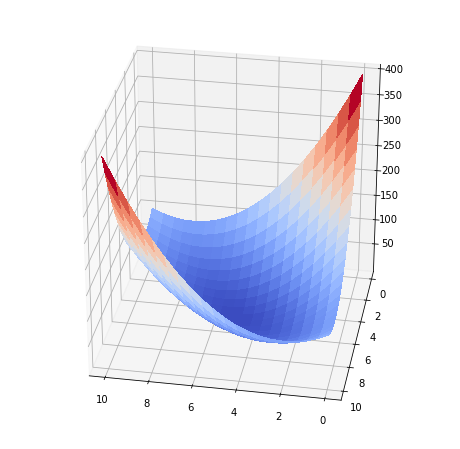Download optimizers.tar and extract optimizers.py.

In :
!head -20 optimizers.py

import copy
import numpy as np
import time
import math
import sys

floatPrecision = sys.float_info.epsilon

class Optimizers():

def __init__(self, all_weights):
'''all_weights is a vector of all of a neural networks weights concatenated into a one-dimensional vector'''

self.all_weights = all_weights

self.scg_initialized = False

# The following initializations are only used by adam.
# Only initializing m, v, beta1t and beta2t here allows multiple calls to adam to handle training
# with multiple subsets (batches) of training data.

In :
import optimizers as opt

def show_trace(fig, x_start, function, function_gradient, function_args, n_epochs, learning_rates):
global x

x = x_start.copy()
optimizer = opt.Optimizers(x)
errors_sgd, weights_sgd = optimizer.sgd(function, function_gradient, function_args,
n_epochs=n_epochs, learning_rate=learning_rates,
verbose=False, save_wtrace=True)

x = x_start.copy()
optimizer = opt.Optimizers(x)
n_epochs=n_epochs, learning_rate=learning_rates,
verbose=False, save_wtrace=True)

x = x_start.copy()
# time.sleep(2)

optimizer = opt.Optimizers(x)
errors_scg, weights_scg = optimizer.scg(function, function_gradient, function_args,
n_epochs=200,
verbose=False, save_wtrace=True)

plt.clf()

xt = weights_sgd
plt.plot(xt[:, 0], xt[:, 1], 'ro-', alpha=0.4, label='SGD')

xt = weights_scg
plt.plot(xt[:, 0], xt[:, 1], 'ko-', alpha=0.4, label='SCG')

plt.plot(xt[:, 0], xt[:, 1], 'go-', alpha=0.2, label='Adam')

plt.contourf(X, Y, Z, 20, alpha=0.3)
plt.axis('tight')

plt.legend()
ipd.clear_output(wait=True)
ipd.display(fig)

In :
fig = plt.figure(figsize=(10, 10))

x_start = np.random.uniform(0, 10, 2)
show_trace(fig, x_start, parabola, parabola_gradient, [center, S],
n_epochs=200, learning_rates=[0.1, 0.5])

ipd.clear_output(wait=True)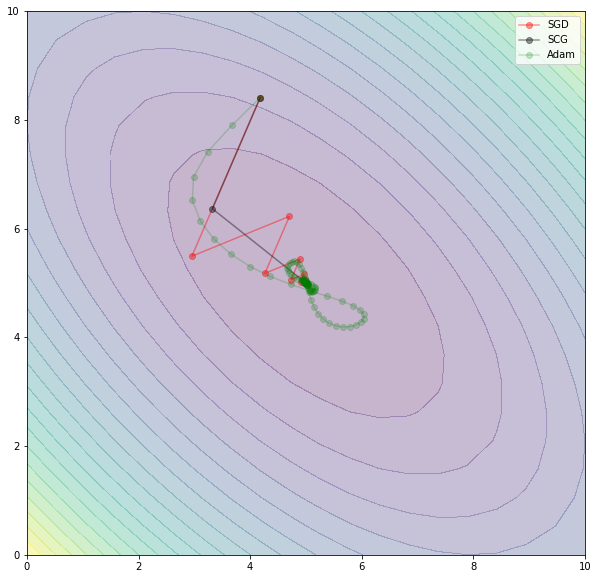Rosenbrock's function is often used to test optimization algorithms. It is

$$f(x,y) = (1-x)^2 + 100(y-x^2)^2$$
In :
def rosen():
v = (1.0 - x)**2 + 100 * ((x - x**2)**2)
return v

g1 = -400 * (x - x**2) * x - 2 * (1 - x)
g2 =  200 * (x - x**2)
return np.array([g1, g2])

In :
n = 10
xmin, xmax = -1,2
xs = np.linspace(xmin, xmax, n)
ys = np.linspace(xmin, xmax, n)
X, Y = np.meshgrid(xs, ys)

both = np.vstack((X.flat, Y.flat)).T
nall = n * n
Z = np.zeros(nall)
for i in range(n * n):
x = both[i]
Z[i] = rosen()
Z.resize((n, n))

fig = plt.figure(figsize=(8, 8))
ax = fig.gca(projection='3d')

# Plot the surface.
surf = ax.plot_surface(X, Y, Z, linewidth=0, antialiased=False,
cmap=plt.cm.coolwarm)
ax.view_init(elev=40., azim=260)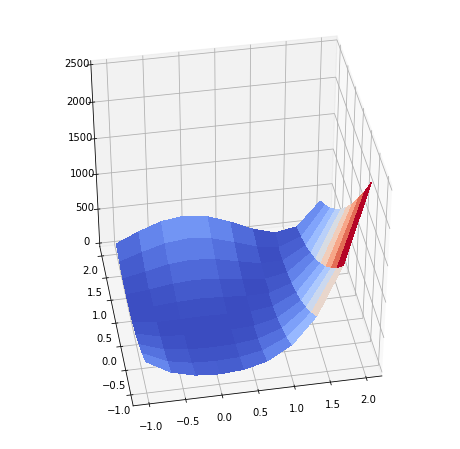In :
fig = plt.figure(figsize=(10, 10))

x_start = np.random.uniform(-1, 2, 2)
show_trace(fig, x_start, rosen, rosen_gradient, [], n_epochs=400,
learning_rates=[0.001, 0.5])

ipd.clear_output(wait=True)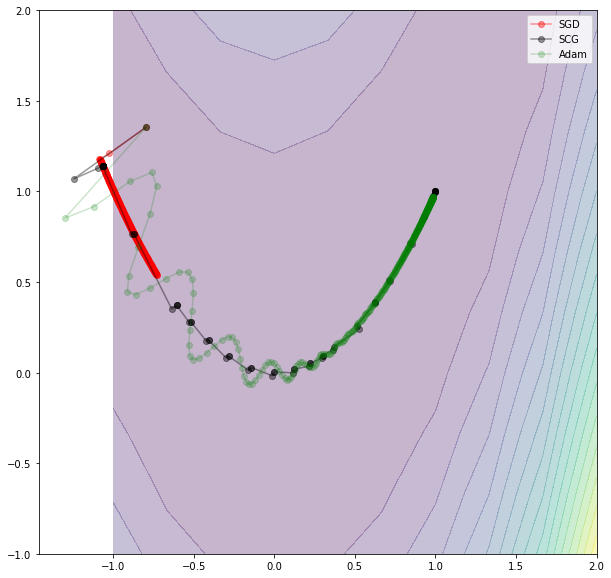In [ ]: